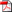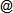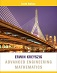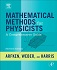ENGR 520School of Engineering
CUA, Spring 2014
Course Information - Description - Textbooks - Topics
Organization - Grading - Schedule, Notes & Assignments
Download SyllabusCourse Information•  Credits: 3.00 •  Schedule: Wednesday, 6:40–9:10pm •  Classroom: McCort-Ward 114 •  Number: 01-LEC (3647) •  Capacity: 35 •  Instructor: Dr. Renť Doursat •  Email: doursatcua.edu •  Phone: (202) 319-5160 •  Office: Pangborn 121 •  Hours: Tuesday & Thursday, 2:30–3:30pm Wednesday, 5:30–6:30pm

Description

The objective of this course is to provide an introduction to the mathematical methods that will be needed in subsequent graduate-level courses in engineering. Emphasis is placed on understanding the concepts for solving first- and second-order differential equations (ODEs), as opposed to extricating an answer from math packages such as Mathematica or MATLAB. The philosophy of understanding the underlying concepts of the math is emphasized throughout the course and is extended to the concept of integration in the complex plane and the origination of the Fourier/Laplace transforms as well as their inverse transforms. Additionally, various special functions are examined including: Gamma, Beta, Bessel, and Legendre (and associated) polynomials. The process of separation of variables in partial differential equations (PDEs) is covered in the three major coordinate systems with application to the Laplace, heat, and wave equations.

Textbooks

• Required TextbookKreyszig, E. (2011) Advanced Engineering Mathematics (10th Edition). Wiley (ISBN: 978-0470458365).
• Optional TextbookArfken, G. B., Weber, H. J. & Harris, F. E. (2012) Mathematical Methods for Physicists (7th Edition): A Comprehensive Guide. Academic Press (ISBN: 978-0123846549).

Topics Covered

This is a tentative list of topics, subject to modification and reorganization.

• First-order ODEs
• Second-order ODEs, Wronskian
• Power series solutions, Bessel functions, Legendre polynomials
• Convergence, regular and essential singularities
• Method of Frobenius, associated Legendre equation
• Integration techniques, complex Z- and W-planes, multi-valued functions and branch cuts
• Cauchy-Riemann equations, deformation of contours, integration about a singularity
• Taylor and Laurent expansions of f(z), Jordan's lemma, Gamma and Beta functions
• Fourier series solutions for periodic functions, Fourier and inverse Fourier transforms
• Laplace and inverse Laplace transforms
• Classification of PDEs
• Separation of variables in Cartesian, cylindrical, and spherical coordinates
• Solution of model equations: Laplace's, wave and heat equations
• Extended discussion of Bessel functions to include development of second solutions, asymptotic representations, Hankel functions (cylindrical and spherical)

Organization
• The use of laptops, tablets, cell phones, cameras, recorders, or other devices is not allowed during any of the meetings (lectures and exams). Bring paper and pens!

• Homework will be assigned after every class (i.e. weekly, except during recess) and due at the beginning of the next class. It will consist of exercises and practice questions intended to test and affirm your knowledge of the course content. While collaboration on the assignments is accepted on the conceptual level, you must write your solutions strictly individually. Late homework will be penalized at a rate of 10% per day.

• Exams will include two midterms (1h15 each) during the semester (see Schedule for tentative dates) and one final. Make-up exams will not be given except in cases of documented emergency.

• Important dates:
• Last day to add/drop courses → Jan 24
• Midterm Exam 1 → Feb 26 (tentative)
• Last day to withdraw → Apr 2
• Midterm Exam 2 → Apr 9 (tentative)
• Final Exam → May 7, 5:45-7:45pm

Both grading policy and scale are subject to change. Failure (< 70) in either the practice component (homework) or the lecture component (exams) will result in an F for the whole course.

 Homework 40% Midterm Exam 1 20% Midterm Exam 2 15% Final Exam 25%

 100 → 90 A, A- 89 → 80 B+, B, B- 79 → 70 C 69 → 0 F

Schedule, Notes & Assignments

This is a tentative schedule, subject to readjustment depending on the time we actually spend in class covering the topics. Please check again regularly. Course notes (if any), assignments and exam solutions are posted on the CUA Blackboard, under "...ENGR 520/Course Documents" and "...ENGR 520/Assignments". Permanent reading assignment: it is assumed that you are familiar with the contents of the course notes of all past meetings.

 Week Dates Topics 1Wed, Jan 15 1. First-Order ODEs:  •  introduction: modeling, classification  •  separable equations → direct integration  •  ax+by+c substitution → reduction to separable  •  exact equations → total differential 2 Wed, Jan 22 •  inexact equations → integrating factor in x, in y, in xmyn  •  balanced equations → function of y/x  •  linear equations → homogeneous, nonhomogeneous  •  Bernoulli equation → reduction to linear 3 Wed, Jan 29 2. Second-Order Linear ODEs:  •  homogeneous → "reduction of order" from one solution  •  homogeneous with cst coeffs → exponential solutions  •  nonhomogeneous with cst coeffs → "undetermined coefficients" 4 Wed, Feb 5 •  nonhomogeneous → "variation of the constant", Wronskian  •  general (nonlinear), missing y, missing x → reduction to first-order 3. Linear PDEs:  •  wave equation (Laplace equation, heat equation)  •  "separation of variables" in Cartesian coordinates → planar waves 5 Wed, Feb 12 •  Laplacian in cylindrical coordinates  •  wave equation in cylindrical coordinates → Bessel equations 4. Power-Series Solutions:  •  infinite series → ratio test, root test  •  power series → radius of convergence  •  solutions of ODEs → index shifting, recurrence relationships 6a Mon, Feb 1712:30-3pm3:30-6pm •  wave equation in spherical coordinates → Legendre equations  •  power series solutions → Legendre polynomials  •  singular points, Frobenius method → Bessel solutions 5. Real Definite Integrals:  •  Gamma function, Beta function  •  Gaussian integral, attenuating factor  •  differentiating under the integral 6b Wed, Feb 19 6. Complex Analysis:  •  complex numbers, polar form, complex roots  •  complex derivative, analytic functions Midterm Review 1 7 Wed, Feb 26 Midterm Exam 1 (1h15) •  Cauchy-Riemann equations  •  complex trigonometric functions, complex logarithm 8 Wed, Mar 5 Class cancelled 9 Wed, Mar 12 Spring recess - no class 10 Wed, Mar 19 7. Complex Integration:  •  path integral, contour integral  •  Cauchy's integral theorem  •  Cauchy's integral formula 11 Wed, Mar 26 8. Complex Series:  •  Taylor series, Laurent series  •  residue integration theorem  •  application to real integrals 12 Wed, Apr 2 9. Fourier Series:  •  period 2π, period 2L  •  even and odd functions Midterm Review 2 13 Wed, Apr 9 Midterm Exam 2 (1h15) •  complex expression  •  solving forced oscillations 14 Wed, Apr 16 10. Fourier Transform:  •  Fourier integral  •  cosine and sine transforms  •  Fourier transform 15 Wed, Apr 23 11. Laplace Transform:  •  basic functions, step, impulse  •  shift, derivative, integral  •  solving ODEs algebraically 16 Wed, Apr 30 Final Review 17 Wed, May 75:45-7:45pm Final Exam (2h)

Course Information - Description - Textbooks - Topics
Organization - Grading - Schedule, Notes & Assignments
Created and maintained by
Renť Doursat
Last update: 2/15/2014